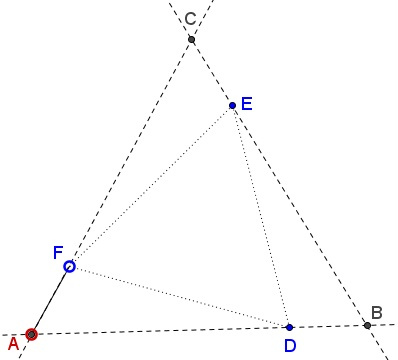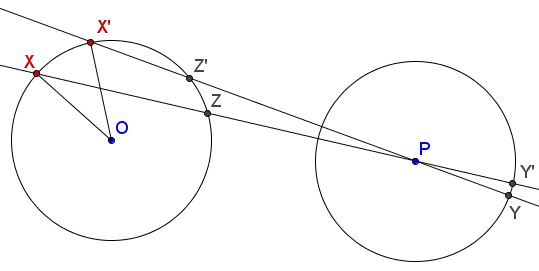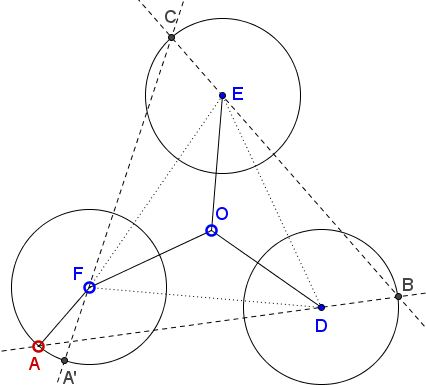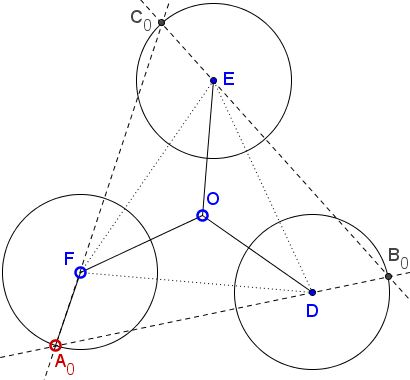# Equilateral Triangle in Equilateral Triangle

### Problem

Given $\Delta ABC,$ and three points on the sides - $D$ on $AB,$ $E$ on $BC,$ and $F$ on $AC.$ $\Delta DEF$ is equilateral, and the lengths of the segments $AF,$ $BD$ and $CE$ are equal.Prove that $\Delta ABC$ is also equilateral.

### Solution

To start with, consider two circles $(O)$ and $(P)$ of the same radius. Let $X$ be an arbitrary point on $(O).$ Join $X$ to $P$ and let $Y$ be the father intersection of $XP$ with $(P).$ Consider mapping $T:\space (O)\rightarrow (P),$ defined with $X\mapsto Y.$ I claim that $T$ is a contraction mapping.

To make that more precise, define metrics $d_O$ and $d_P$ on $(O)$ and $(P),$ respectively as the angular distances between their points, e.g., in the diagram below, $d_{O}(X,X')=\angle XOX'.$ (To be even more precise we may restrict $T$ to the portion of $(O)$ that consists of more distant points of intersection with $(O)$ of lines through $(P).)$Then, with $Z$ and $Z'$ as shown,\begin{align} d_{P}(Y,Y')&=\angle YPY'=\angle XPX'=\frac{1}{2}(\overparen{\rm XX'}-\overparen{\rm ZZ'})\\ &\lt \frac{1}{2}\overparen{\rm XX'}=\frac{1}{2}\angle XOX'\\ &=\frac{1}{2}d_{O}(X,X'). \end{align}

Now, back to the problem at hand.Pick point $A\in (F),$ map it on $B\in (D),$ and from here to $C\in(E),$ and to $A'\in(F).$ All three auxiliary mappings are contractions with the same coefficient $\frac{1}{2}$ such that the mapping of $(O)$ to itself, defined by $A\mapsto A',$ is a contraction with the coefficient $\frac{1}{8}\lt 1.$ According to the Contraction Mapping Theorem there exists a unique fixed point, say, $A_0.$This defines $B_0$ and $C_0$ such that $\Delta A_{0}B_{0}C_{0}$ is equilateral for, otherwise, a rotation by $120^{\circ}$ around $O$ would place $B_0$ on $(F)$ different from $A_0$ and make it another fixed point on $(F).$ So it follows that $A_{0},B_{0},C_{0}$ are obtained from each other with a rotation through $120^{\circ}$ around $O,$ making $\Delta A_{0}B_{0}C_{0}$ equilateral indeed.

Note 1: To apply the Contraction Mapping Theorem we need to make sure that the metric $d_O$ makes $(O)$ a complete metric space. This is left as an exercise.

Note 2: The polygon in the statement need not be a triangle. A similar construction starting with an $n\mbox{-gon},$ $n\gt 3,$ would lead to another regular $n\mbox{-gon}.$

### Acknowledgment

The problem has been posted by Omer Simhi (Haifa, Israel) at the CutTheKnotMath facebook page.

• Angle Trisectors on Circumcircle
• Equilateral Triangles On Sides of a Parallelogram
• Pompeiu's Theorem
• Pairs of Areas in Equilateral Triangle
• The Eutrigon Theorem
• Seven Problems in Equilateral Triangle
• Spiral Similarity Leads to Equilateral Triangle
• Parallelogram and Four Equilateral Triangles
• A Pedal Property in Equilateral Triangle
• Miguel Ochoa's van Schooten Like Theorem
• Two Conditions for a Triangle to Be Equilateral
• Incircle in Equilateral Triangle
• When Is Triangle Equilateral: Marian Dinca's Criterion
• Barycenter of Cevian Triangle
• Excircle in Equilateral Triangle
• Converse Construction in Pompeiu's Theorem
• Wonderful Trigonometry In Equilateral Triangle
• 60o Angle And Importance of Being The Other End of a Diameter
• One More Property of Equilateral Triangles
• Van Khea's Quickie
• Equilateral Triangle from Three Centroids
•